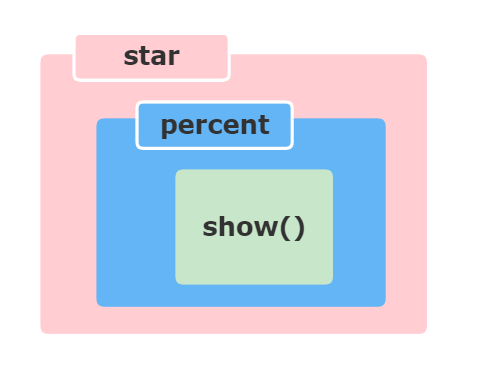# Python中的装饰器

Python装饰器接收一个函数对象，添加一些功能，并返回该函数对象。在本文中，你将了解如何创建装饰器(`decorator`)以及为什么要使用它。

Python 有一个有趣的特性叫做装饰器，可以在不改动现有代码的情况下添加新功能。这也称为元编程（`metaprogramming`），因为程序的一部分试图在编译时修改程序的另一部分。

## 先决条件

• 可以将各种不同的名称绑定到同一个函数对象

``````# 定义一个普通函数
def first(msg):
print(msg)

first("干饭人")   # 调用first函数
second = first   # 将first函数赋值给second变量
second("干饭人")  # 调用second函数
``````

``````干饭人

``````

• 函数可以作为参数传递给另一个函数

``````def add(x):
return x + 1

def lose(x):
return x - 1

def operate(func, x):
result = func(x)
return result
``````

``````operate(add,3)  # 输出：4
operate(lose,3)  # 输出：2
``````
• 一个函数可以返回另一个函数。

``````def is_called():
def is_returned():
print("干饭人")
return is_returned

new = is_called()
new()
``````

``````干饭人
``````

• 闭包

## 装饰器

A decorator is a callable that returns a callable.

``````# 声明第一个函数make_pretty
def make_pretty(func):
def inner():
print("我是make_pretty，我来搞点事情")
func()
return inner

# 声明第二个函数ordinary
def ordinary():
print("我是ordinary，我的内心毫无波澜")
``````

``````ordinary()
``````

``````我是ordinary，我的内心毫无波澜
``````

``````pretty = make_pretty(ordinary)
pretty()
``````

``````我是make_pretty，我来搞点事情

``````

``````@make_pretty
def ordinary():
print("I am ordinary")
``````

``````def ordinary():
print("I am ordinary")
ordinary = make_pretty(ordinary)
``````

## 装饰器参数

``````def divide(a, b):
return a/b

divide(2,5)  # 输出：0.4
divide(2,0)  # 报错 “ZeroDivisionError: division by zero”
``````

``````def smart_divide(func):
def inner(a, b):
print(f"将要计算 {a} 除以 {b}")
if b == 0:
print("分母不能为0！")
return

return func(a, b)
return inner

@smart_divide
def divide(a, b):
print(a/b)
``````

``````print(divide(2,5))
``````

``````将要计算 2 除以 5
0.4
``````

``````print(divide(2,0))
``````

``````将要计算 2 除以 0

None
``````

``````def works_for_all(func):
def inner(*args, **kwargs):
print("I can decorate any function")
return func(*args, **kwargs)
return inner
``````

## 装饰器嵌套

``````def star(func):
def inner(*args, **kwargs):
print("*" * 30)
func(*args, **kwargs)
print("*" * 30)
return inner

def percent(func):
def inner(*args, **kwargs):
print("%" * 30)
func(*args, **kwargs)
print("%" * 30)
return inner

@star
@percent
def show(msg):
print(msg)

show("我是宇宙中心")
``````

``````def printer(msg):
print(msg)
printer = star(percent(printer))
````````````******************************
%%%%%%%%%%%%%%%%%%%%%%%%%%%%%%

%%%%%%%%%%%%%%%%%%%%%%%%%%%%%%
******************************
``````

``````@percent
@star
def printer(msg):
print(msg)
``````

``````%%%%%%%%%%%%%%%%%%%%%%%%%%%%%%
******************************

******************************
%%%%%%%%%%%%%%%%%%%%%%%%%%%%%%
``````

—END